## research papersJOURNAL OFSYNCHROTRONRADIATION
ISSN: 1600-5775
Volume 6| Part 2| March 1999| Pages 105-111

## Combining CXMD and XSW to study magnetic and geometric properties of thin films: Gd/Fe(100)

aHamburger Synchrotronstrahlungslabor HASYLAB, am Deutschen Elektronen-Synchrotron DESY, Notkestrasse 85, D-22603 Hamburg, Germany
*Correspondence e-mail: rolf.treusch@desy.de

(Received 27 August 1998; accepted 22 December 1998)

The magnetic and geometric structure of 1–5 monolayers (ML) of Gd on Fe(100) single crystals has been investigated using synchrotron X-rays between 4 and 8 keV. Choosing a high-flux wiggler beamline made it possible to analyse the magnetic properties by studying the circular X-ray magnetic dichroism (CXMD) and, in addition, to apply the X-ray standing-wave (XSW) technique to elucidate the geometric structure of the overlayers. CXMD reveals that Gd couples antiferromagnetically to the Fe substrate and that 2 ML Gd at 243 K yield a magnetization of ∼22% with respect to the saturation value of a thick Gd foil at 123 K. A magnetic polarization of the Gd pertains even at 308 K. While LEED investigations do not show any long-range lateral order of the Gd film, XSW measurements reveal an ordering of Gd perpendicular to the (100) surface of Fe.

### 1. Introduction

The application of circular X-ray magnetic dichroism (CXMD) to investigate the magnetic properties of solids has been an ever-growing field since its discovery at the experimental station ROEMO at HASYLAB a decade ago (Schütz et al., 1987). While this first study of the circular-polarization dependency of the photoabsorption process close to an edge was purely basic science driven, CXMD has found widespread use because of its importance in studying magnetic properties in pure metals, alloys, multilayers and dilute systems [see, for example, Schütz et al. (1994), and references therein]. The CXMD signal, which is related to the spin-polarized partial density of states populated in an absorption process, provides element-selective site-specific information on the magnetic properties of matter.

In this paper we describe the first successful attempt to measure CXMD of magnetic monolayer films using X-ray wavelengths below 4 Å. This poses a real precision test and challenge for XAS instruments at third-generation insertion-device beamlines such as BW2 at HASYLAB (Drube et al., 1995). However, it also opens up the possibility of adding other powerful X-ray tools, such as X-ray standing waves (XSW) and (spin-dependent) SEXAFS, to study in situ geometric properties of identical adsorbate systems. This is crucial for monolayer studies under ultrahigh-vacuum (UHV) conditions.

At soft' X-ray energies (hν < 2 keV), circular magnetic dichroism has become a standard tool for investigating thin magnetic films in the monolayer range. While for soft X-rays the magnetic part of the absorption coefficient is of the order of 10% (e.g. at the L-absorption edges of 3d metals or the M-edges of rare earths), the CXMD effects at 7–8 keV (3d-metal K-edges and rare-earth L-edges) are only about 1% with respect to the absorption coefficient. Also, the decreased photoabsorption cross sections and the increased bulk sensitivity at higher photon energies result in a reduced signal-to-noise ratio. However, as demonstrated in this work, the availability of intense circularly polarized synchrotron radiation, e.g. by using asymmetrical wigglers or helical undulators (Goulon et al., 1987; Yamamoto & Kitamura, 1987; Pflüger & Heintze, 1990) or quarter-wave plates (Giles et al., 1994), has made these experiments feasible even for monolayer coverages.

The use of photon energies above 2 keV allows the application of the X-ray standing-wave (XSW) technique (Zegenhagen et al., 1990; Zegenhagen, 1993) to investigate the geometric properties of the system. Since the initial work of Cowan et al. (1980) and the subsequent application of synchrotron radiation by Materlik & Zegenhagen (1984), XSW has become a valuable tool for studying the structural properties of crystal surfaces and adsorbate systems. Whereas diffraction methods, such as LEED, probe the two-dimensional long-range order, XSW is sensitive to short-range order. The local geometry of adsorbates on crystalline substrates can be determined, even if no long-range order is present. While XSW studies in gaseous or liquid environment or within solids need a short X-ray wavelength to overcome absorption, longer wavelengths can be used for studies in the UHV environment, which is natural for most surface studies. Thus, XSW can benefit from the λ2 dependence of the width of the single-crystal reflection curve. Measurements can even be carried out around θB = 90° where the angular reflection range becomes maximum. This normal-incidence geometry (NIXSW) (Woodruff et al., 1987) is well suited for investigating metal crystals.

The combination of CXMD and NIXSW is applied for Gd/Fe(100) as a test system because its magnetic properties have also been investigated with spin-polarized Auger electron spectroscopy (Taborelli et al., 1986), spin- and angle-resolved photoemission (Carbone & Kisker, 1987), soft X-ray magnetic circular dichroism (Kachel et al., 1994) and theoretically (Blügel, 1988). Fe is the most suitable substrate for remanent magnetization, and the Gd CXMD signal is comparably large due to seven unpaired 4f electrons. It has been shown earlier that Gd couples antiferromagnetically to the Fe substrate and that the magnetization decreases with increasing film thickness. A Curie temperature of TC ≃ 800 K for 1 ML Gd/Fe(100) has been found (Taborelli et al., 1986) which is about 500 K above the corresponding value for bulk Gd. Whereas the magnetic properties have been extensively studied, the ordering of Gd on Fe is still an open question. It is observed that the Fe (1 × 1) LEED pattern vanishes at Gd coverages of ∼2 ML indicating that no superstructure is formed.

### 2. Experiment

The experiment was performed at the high-flux wiggler beamline BW2 at HASYLAB (Drube et al., 1995) using focused monochromatic synchrotron radiation from the DORIS III storage ring. For the XSW measurements at a photon energy of ∼4.3 keV, a symmetric wiggler (Pflüger & Gürtler, 1990) was used resulting in a photon flux of 5 × 1012 s−1 at the sample with an energy width ΔE/E = 2 × 10−4 for the Si(111) double-crystal monochromator in use. The focal spot at the sample was 1 × 1 mm2. Circularly polarized light for the CXMD measurements in the photon energy range 7–8 keV was obtained from an asymmetric hybrid wiggler (Pflüger & Heintze, 1990). For this device the monochromated photon flux accepted ∼0.15 mrad off-plane amounts at 8 × 1010 s−1 with 50% circular polarization, spot size and energy resolution as above.

Sample preparation was performed in situ in a multipurpose UHV chamber (Drube et al., 1992). The Fe crystals were cleaned by repeated cycles of argon sputtering (1–1.5 kV, 5–20 µA cm−2, grazing incidence) and annealing at 823–873 K. Surface cleanliness was checked by LEED and AES. The Gd films were evaporated using an electron-beam evaporator with a Gd rod target and typical evaporation rates of 0.2 ML min−1 at a base pressure of 8 × 10−10 mbar.

### 3. CXMD studies

In general, an absorption measurement with circularly polarized X-rays is described by an absorption coefficient μ with two contributions – a spin-independent part μ0 and a spin-dependent part μc. For a detailed quantitative analysis of CXMD spectra, the spin-dependent absorption coefficients have to be obtained from (relativistic) spin-polarized self-consistent band-structure calculations (Ebert et al., 1990; Carra et al., 1991). In the following we give a commonly used simplified qualitative interpretation. In a first approximation, μc is related to the spin-dependent density of unoccupied states through Fermi's Golden Rule,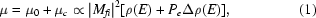where Mfi is the transition matrix element, ρ is the total density of states and Δρ is the difference in the density of states for majority and minority spins. The spin polarization, Pe, of the transferred photoelectron is known as the Fano parameter. It is a measure of the spin transfer from the circularly polarized photon to the electron via spin–orbit interaction (Fano, 1969). In an atomic picture using a vector-coupling model, the Fano parameter is obtained by a simple arithmetic weighting of the transition to the |lsmlms| final states with their Clebsch–Gordan coefficients. The CXMD process for the photoabsorption at the L2-edge of Gd is sketched in Fig. 1.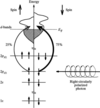Figure 1 Schematic illustration of the Δl = +1 dipole transitions from the Gd 2p1/2 level into empty d states for the absorption of a right circularly polarized photon. The magnetic orientation is along the propagation direction of the photon, i.e. parallel to its spin. Due to the exchange interaction, the d bands for spin ↑ and spin ↓ are energetically split (differences in the density of states are neglected). The absorption from a spin ↓ ground state to a spin ↓ final state is preferred with a ratio of 75%/25% resulting in a Fano parameter Pe = −0.5. Likewise, the absorption of a left circularly polarized photon results in a preferred spin ↑ to spin ↑ transition with the same 75%/25% ratio relative to the corresponding spin ↓ to spin ↓ transition.

To compare data obtained in different experiments, the results are commonly given as the normalized spin-dependent absorption coefficient,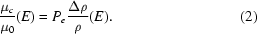μc/μ0 can be derived from the absorption coefficients μ± = μ0 ± μc for the two opposite directions of circular polarization (or magnetization) by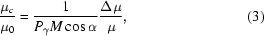where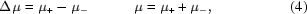Pγ is the degree of circular polarization at the sample, M is the sample magnetization relative to the saturation value and α is the angle between sample magnetization and photon spin, i.e. its k vector.

In this experiment, an Fe(100)5.7 at.%Si crystal was used for the CXMD measurements. A small admixture of Si is necessary because pure Fe crystals exhibit a phase transition from b.c.c. to f.c.c. structure at 1183 K (αγ transition) and thus cannot be grown from the melt. By alloying Si to Fe, the αγ phase transition is suppressed (Hansen, 1958) and larger crystals can be Czochralski-grown. The crystal was shaped like a picture frame to avoid demagnetizing magnetic stray fields (Zalm et al., 1979; Alvarado et al., 1982). It was magnetized parallel to its (100) surface and an angle α = 50° between magnetization and photon spin was used. Magnetic saturation of the Fe was obtained with pulsed currents of 10–12 A through self-supporting Ta coils of four turns each wrapped around two bars of the picture frame (Fig. 2).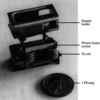Figure 2 The sample holder with an Fe(100) picture frame' crystal and the self-supporting Ta coils used for magnetization and heating. The outer dimensions of the crystal are 15 × 15 mm2. All four bars of the crystal are parallel to 〈100〉 directions, i.e. all surfaces are {100} surfaces. The stainless holder fits to the sample transfer mechanism and manipulator.

In the absorption measurement the CXMD signal was derived from the total electron yield (TEY) of the sample which is, in a good approximation, proportional to the absorption coefficient μ. At fixed photon helicity, the direction of the sample magnetization was reversed several times at each point of the photon energy scan. Two absorption spectra – one for each direction of magnetization – were recorded with an accumulation time of 20–60 s per magnetization direction and data point. Dichroism data for Fe were taken at a sample temperature of 308 K (TC = 1043 K). Since the Curie temperature for Gd is considerably lower (TC,bulk = 293 K), the sample was cooled to 243 K in the Gd/Fe experiment. The temperature was monitored over an extended time until stable conditions were reached. During the CXMD measurements, the thermocouple was removed from the sample to avoid any distortion of the small magnetic signals of 10–100 fA (TEY) due to leak currents. The CXMD measured at the Fe K-absorption edge (7112 eV) of the picture-frame crystal (Fig. 3) was compared with data obtained in transmission for an Fe foil1 to verify magnetic saturation. The maximum of μc0 was (0.9 ± 0.1)% for the crystal and (0.8 ± 0.1)% for the foil.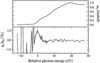Figure 3 CXMD signal of the clean Fe(100) picture frame at the Fe K-edge obtained from the TEY. The photon energy is given relative to the inflection point of the absorption edge (7112 eV) shown in the upper panel for reference. The sample temperature was 308 K. The normalized spin-dependent absorption coefficient μc/μ0 takes into account the photon polarization (50%) and the angle between sample magnetization and photon spin (50°). The broken line is meant as a guideline for the eye.

For the CXMD measurements of the Gd monolayers we preferred the Gd L2-absorption edge (7930 eV) to the L3-edge (7243 eV) to avoid any disturbance from the underlying Fe K-edge modulations. The Gd CXMD spectra at the L2-absorption edge were recorded for 2 ML Gd/Fe at (243 ± 5) K (Fig. 4) and (308 ± 3) K (15 K above TC of bulk Gd) (Fig. 5) and for 5 ML Gd/Fe at (243 ± 5) K. Compared with the reference data for Gd and Fe foils, a sign reversal of the Gd CXMD signal indicates antiferromagnetic coupling of Gd to the Fe substrate. The results are summarized in Table 1. With respect to the saturation value of the Gd foil at 123 K, the normalized spin-dependent absorption μc/μ0 is (22 ± 5)% for 2 ML Gd at 243 K, (14 ± 4)% at 308 K and (13 ± 3)% for 5 ML Gd at 243 K sample temperature. The results for 2 ML Gd show a clearly enhanced Curie temperature with respect to the bulk material. The averaged magnetization of the 5 ML Gd is about 60% of the 2 ML Gd film, showing that the upper Gd layers are less magnetized. This is due to a stronger magnetic coupling of Gd to the Fe substrate compared with the coupling within the Gd layers (while the magnetic coupling between the Gd 4f electrons and the Fe 3d electrons is indirect via the overlap with the Gd 5d wave function, the Gd–Gd coupling is induced by the weaker 4f–5d–5d–4f overlap).

 Table 1Summary of the CXMD results
 The relative error of the normalized spin-dependent absorption coefficient μc/μ0 is ∼20%. This error is dominated by the uncertainty in the determination of Pγ, the degree of circular polarization at the sample.
Sample Signal Temperature (K) Maximum of μc/μ0 (%)
Fe picture-frame crystal TEY 308 0.9
Fe foil Transmission 298 0.8
2 ML Gd/Fe TEY 243 0.94
2 ML Gd/Fe TEY 308 0.60
5 ML Gd/Fe TEY 243 0.57
Gd foil Transmission 123 4.2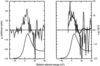Figure 4 CXMD data for 2 ML Gd/Fe(100) at the Gd L2-edge, obtained from the TEY at a sample temperature of 243 K. The photon energy is referenced to the inflection point of the absorption edge (7930 eV) which is displayed in the lower part of the two panels. The normalized spin-dependent absorption coefficient μc/μ0 is shown in the upper right panel. For comparison, the unscaled value, μc, is also shown (upper left panel) because there the CXMD is better visible. The thick line shows the result of a transmission measurement of a Gd foil at 123 K. It is scaled by a factor of −0.22. In the evaluation of μc/μ0 the photon polarization (50%) and the angle between sample magnetization and photon spin (50°) have been taken into account.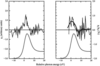Figure 5 As Fig. 4but for a sample temperature of 308 K. The transmission data for a Gd foil at 123 K (thick line in the upper panel) are scaled by a factor of −0.13.

### 4. XSW studies

For a Bragg reflection in a crystal, the interference of the incident and reflected beam results in a standing wave field (von Laue, 1960; Batterman & Cole, 1964). The periodicity of this X-ray standing wave (XSW) is given by the separation dH of the corresponding diffraction planes. By tuning the photon energy or the incidence angle of the crystal in the vicinity of the Bragg angle θB, the phase difference between the incoming wave E0 and reflected wave EH, i.e. the position of the nodes and antinodes of the XSW, can be varied. As θ is decreased through θB (or the photon energy E is decreased through EB), the phase of the XSW shifts continuously from 0 (antinodes at the diffraction planes) to π (nodes at the diffraction planes) in the direction of the reciprocal lattice vector H (Fig. 6).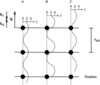Figure 6 Dependence of the phase of an X-ray standing wave field on the incidence angle θ (or the photon energy). Displayed is the secondary yield I which equals the scaled intensity of the electric field in the crystal. A, high-angle flank of the reflection curve (θ > θB or E > EB); B, peak of the reflection curve; C, low-angle flank of the reflection curve (θ ≃ θB or E ≃ EB). For simplicity, it is assumed that R = 0.5, ν = 0 for A, R = 1, ν = π/2 for B and R = 0.5, ν = π for C [cf. equation (5)]. Note the shift of the nodes/antinodes.

Within the dipole approximation, the response of the atoms (e.g. fluorescence, photoemission or Auger electron emission) to this XSW is proportional to the field intensity at the corresponding position. Measuring this element-specific secondary yield while the crystal angle is tilted through the Bragg reflection (or the photon energy is varied correspondingly) allows the determination of the respective atom position.

This yield I(θ) can be written as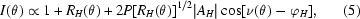where θ is the reflection angle, RH is the reflectivity, ν is the phase of the standing wave field and P is the polarization factor of the incident X-rays [P = 1 for σ-polarization and P = cos (2θB) for π-polarization]. AH and φH denote the phase and the amplitude of the H-Fourier component of the atomic density distribution describing the emitted secondary yield. While the coherent position,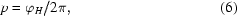gives the distance of the atoms perpendicular to the diffracting net planes in units of dH, the coherent fraction,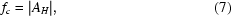can be understood in the single-position case as the ordered fraction of the selected atoms at this site.

In our case the Bragg angle of the sample was chosen close to 90°. This normal-incidence XSW has certain advantages: for θB = 90° (hν = 4.326 keV) the angular (Darwin) width of the Fe(200) reflection reaches its maximum of 0.8°. This makes the reflection and the modulation of the corresponding site-specific secondary yield signal less sensitive to crystal imperfections. Thus a great potential of the NIXSW technique is the investigation of metal crystals. The wide angular acceptance simplifies studies on these crystals which are generally less perfect than semiconductor crystals. That holds, for example, for Cu (Materlik et al., 1985; Woodruff et al., 1987) and Fe (this work). Furthermore, a focused beam and a dispersive set-up (monochromator, sample) can be used without a significant degradation of the position information.

In our experiment the photon energy was scanned to shift the XSW phase while the Bragg angle of the sample was fixed. In this case we used a pure Fe(100) crystal (no Si alloyed). This crystal was grown using the `strain anneal' technique (Landize, 1971) which limited its size to a diameter of ∼5 mm. Due to this size limitation, such a crystal would have been inappropriate for the CXMD measurements. Fig. 7shows the NIXSW results for the (200) reflection of the clean Fe sample (no overlayer). The reflection curve R of the crystal was measured via the photocurrent of a Ta plate hit by the reflected beam which had an offset of 2–4° from the direct beam. As a yield signal, the TEY (sample drain current) was chosen. The coherent fraction, fc = 0.98 ± 0.03, obtained from these data indicates a good crystalline quality of the sample. This is also supported by X-ray topography images (not shown) yielding a mosaic spread of less than 0.02°. However, the resulting position of p = 1.09 ± 0.03 deviates significantly from the expected value of 1.00. This behaviour is the subject of further investigations.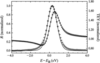Figure 7 XSW results for the Fe(100) crystal: normalized Fe(200) reflection R (○) and modulation of the TEY (×) at θB = 89° (E = 4327 eV). The fit to the data gives p = 1.09 ± 0.03 and fc = 0.98 ± 0.03.

To study the Gd overlayer, we chose the MNN Auger electrons (∼800–1100 eV) as an adsorbate-specific signal. Energy-distribution curves were recorded with a hemispheric electron-energy analyser. The background-corrected Auger signal was integrated for kinetic energies in the range 800–900 eV. From the resulting yield for 1 ML Gd/Fe(100) (Fig. 8) we obtain a coherent position p = 1.12 ± 0.03 and a coherent fraction fc = 0.45 ± 0.05.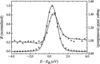Figure 8 XSW data for 1 ML Gd/Fe(100): normalized Fe(200) reflection R (○) and modulation of the Gd MNN Auger yield (×) at θB = 88° (E = 4329 eV) resulting in p = 1.12 ± 0.03 and fc = 0.45 ± 0.05.

Using the result for Fe as a reference for the topmost substrate layer, a relative position of p = 1.03 ± 0.04 is obtained for Gd. Given the covalent atomic radii of 1.24 Å and 1.79 Å for Fe and Gd, respectively, this indicates a mixture of Gd atoms sitting on top (p = 1.11) and at a bridge site (p = 0.86) (Fig. 9). This is supported by the measured coherent fraction of 0.45 considering that the maximum value in this case is fc = 0.71 for two equally populated positions in a completely ordered Gd overlayer. The fourfold coordinated hollow site (p = 0.57), on the other hand, is incompatible with our data and can thus be ruled out.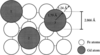Figure 9 Assumed positions of Gd on the Fe(100) surface. 1, on top, p = 1.11; 2, bridge site, p = 0.87; 3, hollow site, p = 0.57.

To summarize, our findings suggest that the magnetic polarization of Gd on Fe(100) crystals does not depend on a unique position, although we cannot fully rule out that the magnetic coupling is dominated by either one of the two sites.

### 5. Conclusions

Using X-ray energies between 4 and 8 keV we investigated Gd monolayer films on Fe(100) crystals using the circular X-ray magnetic dichroism and the X-ray standing-wave techniques. In both methods it is possible to discriminate substrate- and adsorbate-specific information. The combination of the two techniques offers new perspectives for the in situ characterization of electronic and structural properties of thin magnetic films on crystalline substrates. It allows an element-specific study of temperature- and coverage-dependent correlations between magnetic and geometric properties of the substrate and its adsorbate(s). Due to the high information depths in the X-ray range, the study of buried layers and multilayers is also feasible.

It has been demonstrated that, even in the X-ray range, CXMD measurements on monolayer samples can be performed. The stability of third-generation synchrotron radiation sources in conjunction with dedicated optimized undulators, beamlines and instrumentation now opens the way to a wide research field.

### Footnotes

1The measurements were performed at BW2 in collaboration with P. J. Fischer, C. Detlefs, S. Dürndorfer and J. Diehl, University of Augsburg, Germany.

### Acknowledgements

We would like to thank H.-G. Schmidt, T. Dose and U. Brüggmann for assistance with setting up the experiments. The support of R. Dähn and M. Griebenow during the experiments and the cooperation with P. J. Fischer were much appreciated.

### ReferencesJOURNAL OFSYNCHROTRONRADIATION